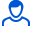Content: teorver_5567.doc (28.50 KB)

Positive responses: 0
Negative responses: 0

Refunds: 05567. x̅=4, n=100, σ=10. Determine the confidence interval for estimating with reliability γ=0.97 the unknown mathematical expectation a of the normally distributed feature X of the general population, if the sample mean x̅в=4, the sample size n=100 and the general standard deviation σ=10 are known.
Detailed solution. Decorated in Microsoft Word 2003 (Quest decided to use the formula editor)
No feedback yet